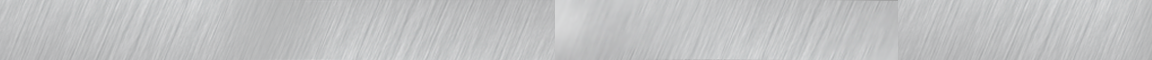# TECH MATTERSbailey Metal Processing Limited - Products

• ## r-Value and Related Parameters

by Esther Mar | Nov 16, 2017
• An important parameter which was not discussed in the module on tensile testing, because it is not a direct result of a standard tensile test, is the r-value. It is, however, obtained using a tensile test coupon in a variation of the test.
• The r-value, the “plastic strain ratio” of sheet metal intended for deep-drawing applications, is a measure of the resistance to thinning or thickening when subjected to either tensile or compressive forces in the plane of the sheet i.e. it is the ability to maintain thickness as the material is drawn.
• The r-value is the ratio of the true width strain to the true thickness strain at a particular value of length strain (strains of 15-20% are commonly used for determining the r-value of low carbon sheet steel).
• Due to the difficulty in measuring thickness changes with sufficient precision, in practice an equivalent relationship is commonly used, based on length and width strain measurements.
• Specifically the tensile coupon is pulled to a specified strain, the machine is stopped and unloaded, and a calculation is made using the original and final lengths and the original and final widths of the coupon.
• The r-value is often different in the longitudinal, transverse and 45° directions and these relationships are different for different sheet products
• A material that exhibits unequal properties when tested in different directions is said to be anisotropic. Anisotropy is caused by mechanical fibering due to the directionality of the rolling process. Anisotropy is, more importantly, also caused by preferred crystallographic orientation or “texture” in the steel.
• Using the r-values in the three directions, two other important parameters are calculated.
• Delta r (∆ r) is called the “planar anisotropy parameter” in the formula:

∆ r = (r0 + r90 - 2r45)/2

This is an indicator of the ability of a material to demonstrate a non-earing behavior (lower is better).

• rm, also called r-bar (i.e. r average) is the “normal anisotropy parameter” in the formula:

rm =(r0 + r90 + 2r45)/4

This is an excellent indicator of the ability of a material to be deep drawn (higher is better).

• A combination of high rm and ∆ r provides optimum drawability.Anisotropy values are evaluated from this tensile sheet specimen.

A strain of 15% is imposed on the specimen.Planar anisotropy is determined with the aid of specimens

cut at various angles to the rolling direction.  Normal anisotropy

is equal to the average of anisotropy values around the surface of the sheet.# ARCHIVE

Do you an idea, feedback or remark for our blog? Drop us a line in the comment section or email us at info@baileymetalprocessing.com.